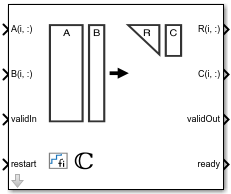Main Content

# Complex Burst QR Decomposition

QR decomposition for complex-valued matrices

• Library:
• Fixed-Point Designer HDL Support / Matrices and Linear Algebra / Matrix Factorizations

•## Description

The Complex Burst QR Decomposition block uses QR decomposition to compute R and C = Q'B, where QR = A, and A and B are complex-valued matrices. The least-squares solution to Ax = B is x = R\C. R is an upper triangular matrix and Q is an orthogonal matrix. To compute C = Q', set B to be the identity matrix.

## Ports

### Input

expand all

Rows of matrix A, specified as a vector. A is an m-by-n matrix where m ≥ 2 and n ≥ 2. If B is single or double, A must be the same data type as B. If A is a fixed-point data type, A must be signed, use binary-point scaling, and have the same word length as B. Slope-bias representation is not supported for fixed-point data types.

Data Types: `single` | `double` | `fixed point`
Complex Number Support: Yes

Rows of matrix B, specified as a vector. B is an m-by-p matrix where m ≥ 2. If A is single or double, B must be the same data type as A. If B is a fixed-point data type, B must be signed, use binary-point scaling, and have the same word length as A. Slope-bias representation is not supported for fixed-point data types.

Data Types: `single` | `double` | `fixed point`
Complex Number Support: Yes

Whether inputs are valid, specified as a Boolean scalar. This control signal indicates when the data from the A(i,:) and B(i,:) input ports are valid. When this value is 1 (`true`) and the value at ready is 1 (`true`), the block captures the values on the A(i,:) and B(i,:) input ports. When this value is 0 (`false`), the block ignores the input samples.

After sending a `true` validIn signal, there may be some delay before ready is set to `false`. To ensure all data is processed, you must wait until ready is set to `false` before sending another `true` validIn signal.

Data Types: `Boolean`

Whether to clear internal states, specified as a Boolean scalar. When this value is 1 (`true`), the block stops the current calculation and clears all internal states. When this value is 0 (`false`), and the validIn value is 1 (`true`), the block begins a new subframe.

Data Types: `Boolean`

### Output

expand all

Rows of the economy size QR decomposition matrix R, returned as a scalar or vector. R is an upper triangular matrix. R has the same data type as A.

Data Types: `single` | `double` | `fixed point`

Rows of the economy size QR decomposition matrix C=Q'B, returned as a scalar or vector. C has the same number of rows as R. C has the same data type as B.

Data Types: `single` | `double` | `fixed point`

Whether the output data is valid, returned as a Boolean scalar. This control signal indicates when the data at output ports R(i,:) and C(i,:) is valid. When this value is 1 (`true`), the block has successfully computed the R and C matrices. When this value is 0 (`false`), the output data is not valid.

Data Types: `Boolean`

Whether the block is ready, returned as a Boolean scalar. This control signal indicates when the block is ready for new input data. When this value is 1 (`true`), and the validIn value is 1 (`true`), the block accepts input data in the next time step. When this value is 0 (`false`), the block ignores input data in the next time step.

After sending a `true` validIn signal, there may be some delay before ready is set to `false`. To ensure all data is processed, you must wait until ready is set to `false` before sending another `true` validIn signal.

Data Types: `Boolean`

## Parameters

expand all

The number of rows in matrices A and B, specified as a positive integer-valued scalar.

#### Programmatic Use

 Block Parameter: `m` Type: character vector Values: positive integer-valued scalar Default: `4`

The number of columns in input matrix A, specified as a positive integer-valued scalar.

#### Programmatic Use

 Block Parameter: `n` Type: character vector Values: positive integer-valued scalar Default: `4`

The number of columns in input matrix B, specified as a positive integer-valued scalar.

#### Programmatic Use

 Block Parameter: `p` Type: character vector Values: positive integer-valued scalar Default: `1`

## Tips

Use `fixed.getQRDecompositionModel(A,B)` to generate a template model containing a Complex Burst QR Decomposition block for complex-valued input matrices `A` and `B`.

## See Also

### Functions

Introduced in R2019b

## SupportGet trial now